理解TSNE算法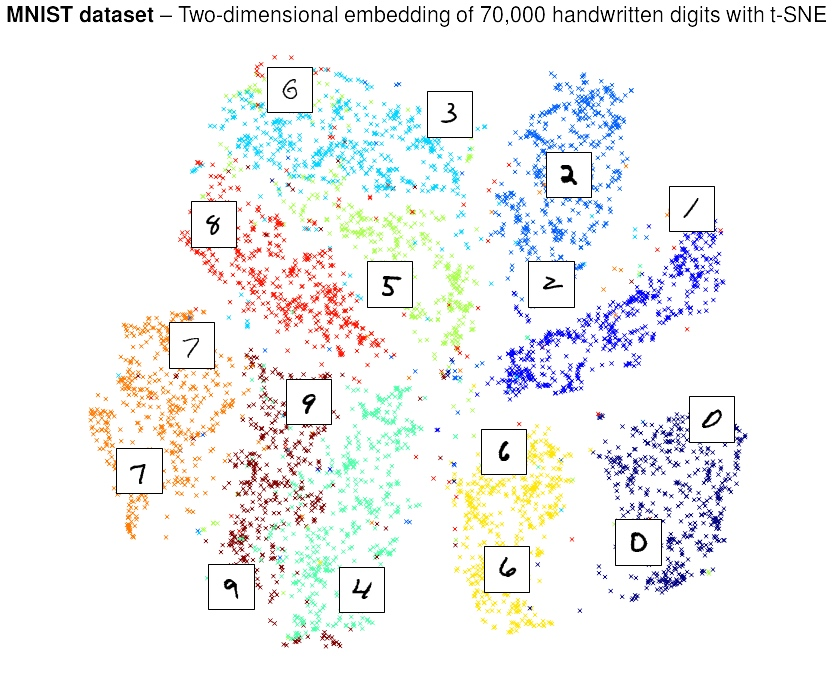t-SNE 是一種數據可視化工具，它可以將高維數據降維到2-3維以用於畫圖，局部相似性被這種embedding所保留。

t-SNE把原空間的數據點之間的距離轉換為高斯分佈概率，如果兩點在高維空間距離越近，那麼這個概率值越大。注意到高斯分佈的這個標準差\$sigma_i\$ 對每個點都是不同的，這也是算法的創新點之一，因為理論上空間不同位置的點的密度是不同的，條件概率如此計算，

\$\$p_{j|i} = frac{exp{(-d(boldsymbol{x}_i, boldsymbol{x}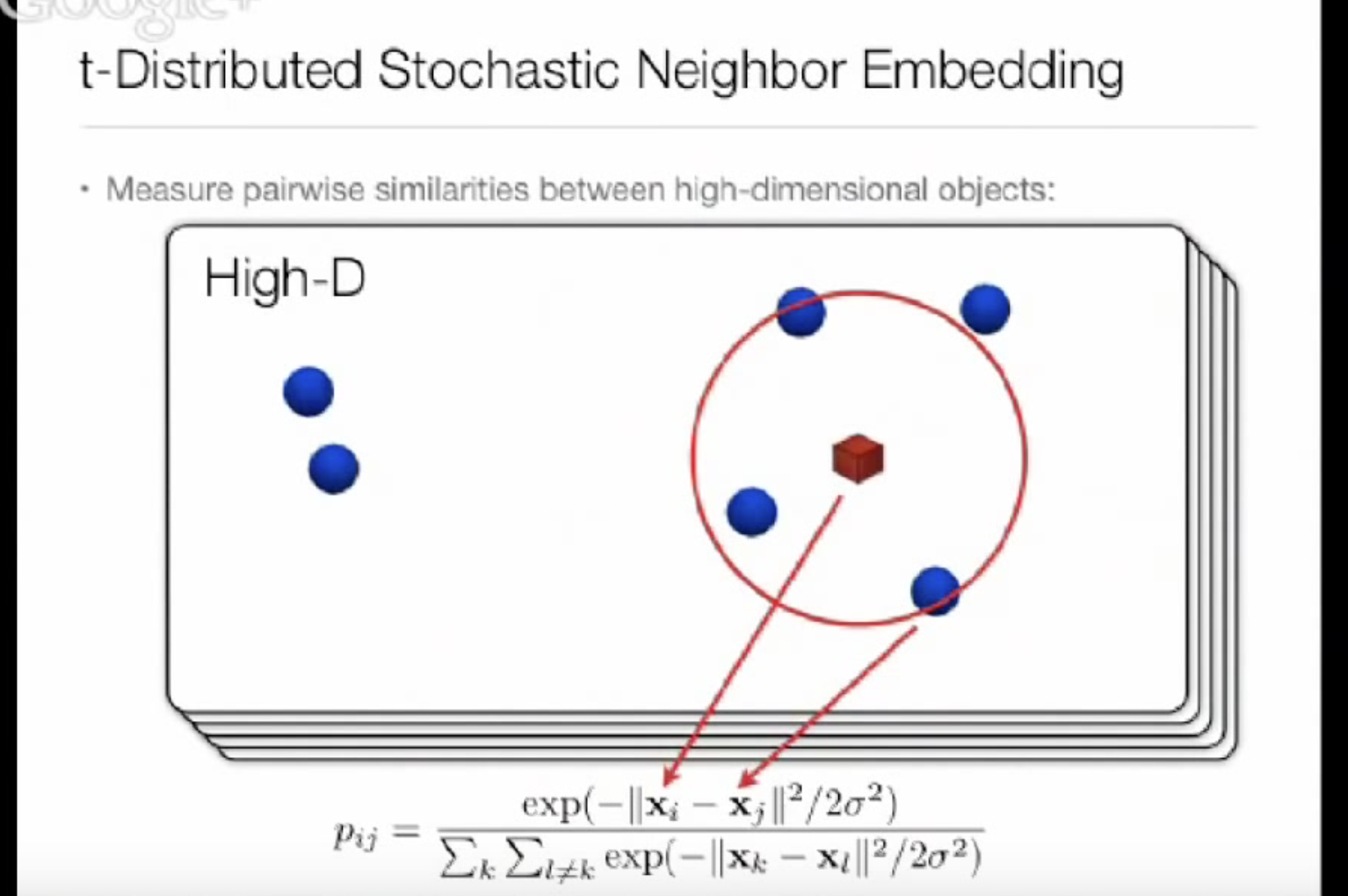\$\$p_{ij} = frac{p_{j|i} + p_{i|j}}{2N}.\$\$

\$\$q_{ij} = frac{(1 + ||boldsymbol{y}_i – boldsymbol{y}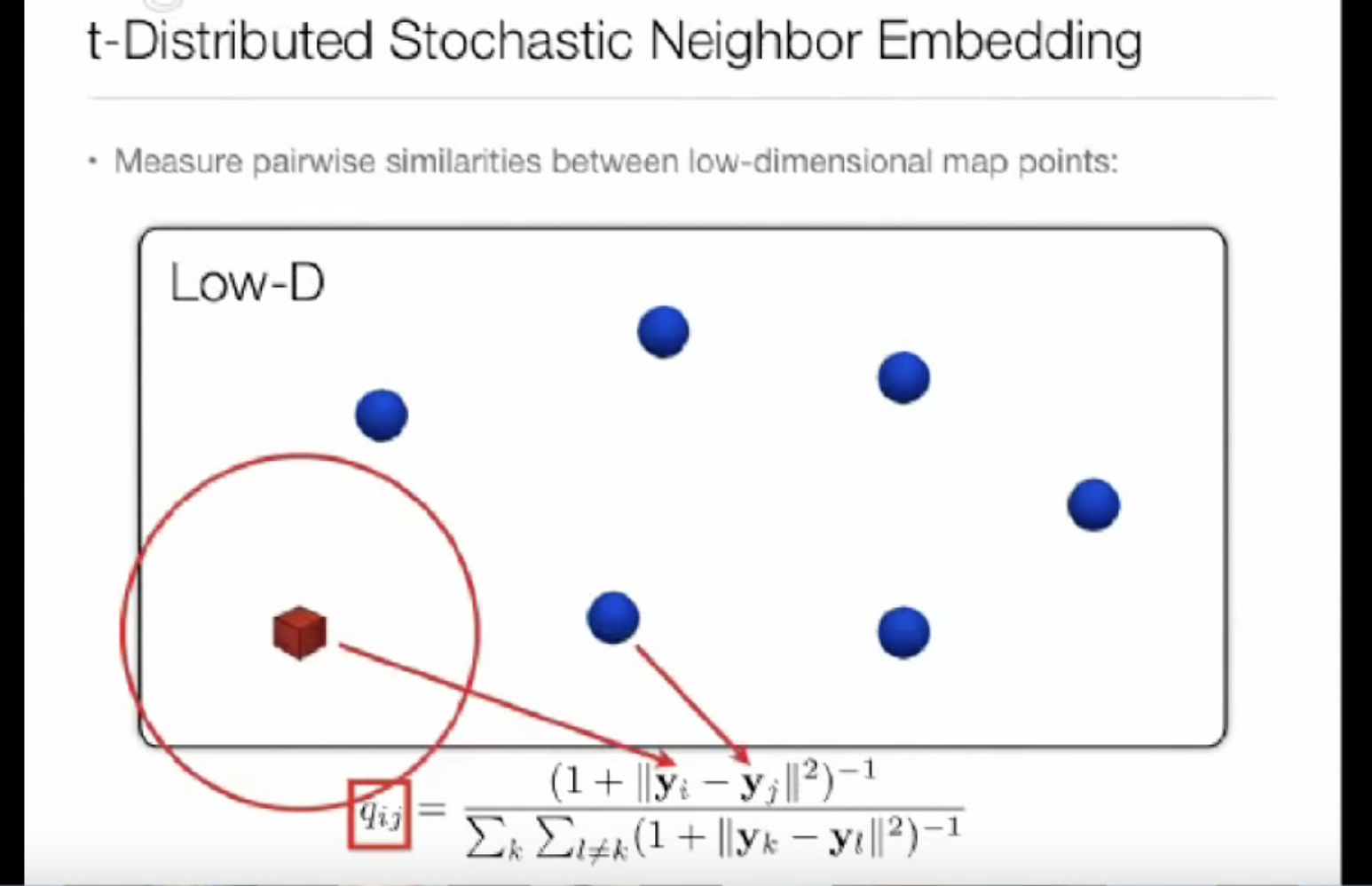\$\$KL(P|Q) = sum_{i neq j} p_{ij} log frac{p_{ij}}{q_{ij}}\$\$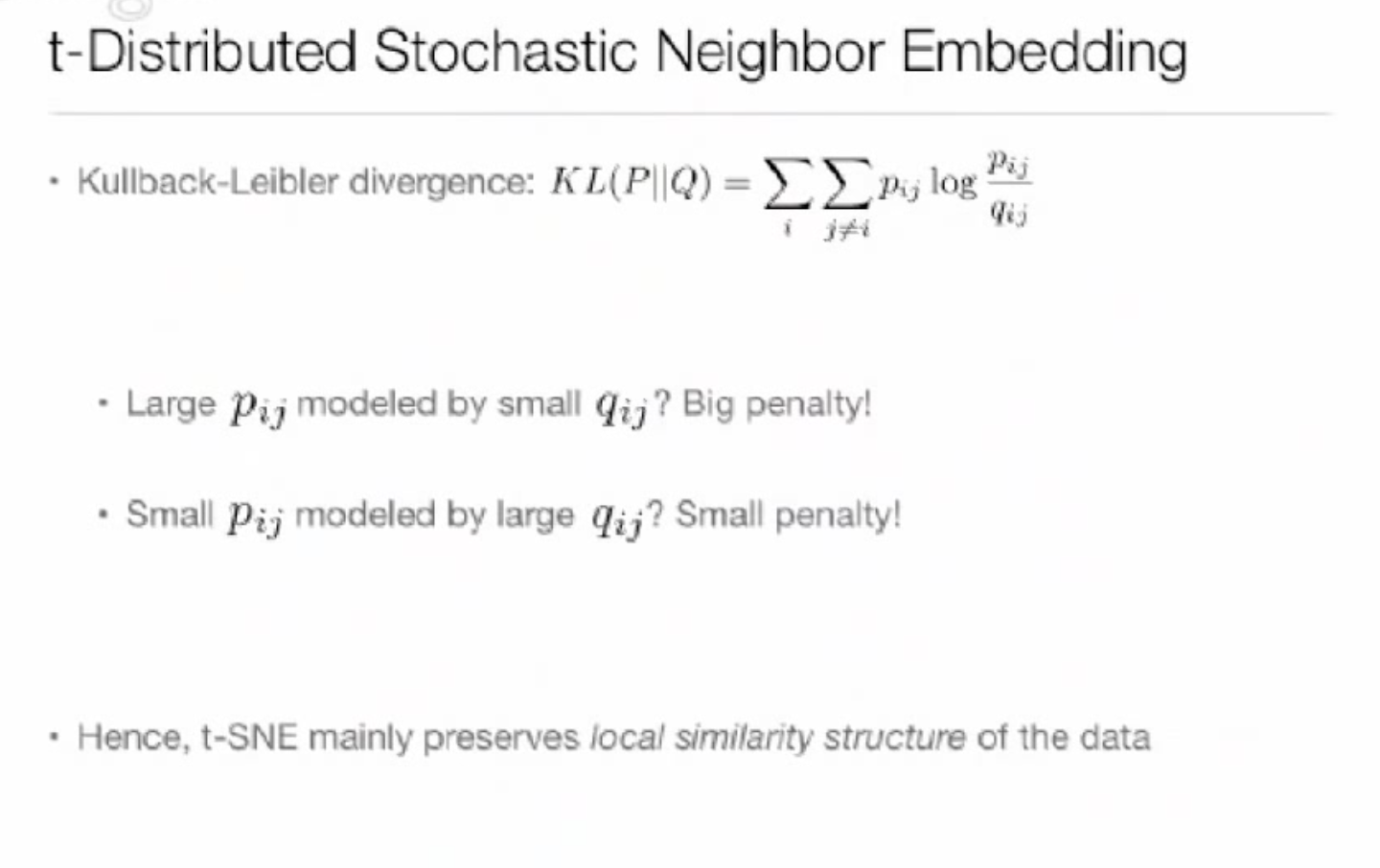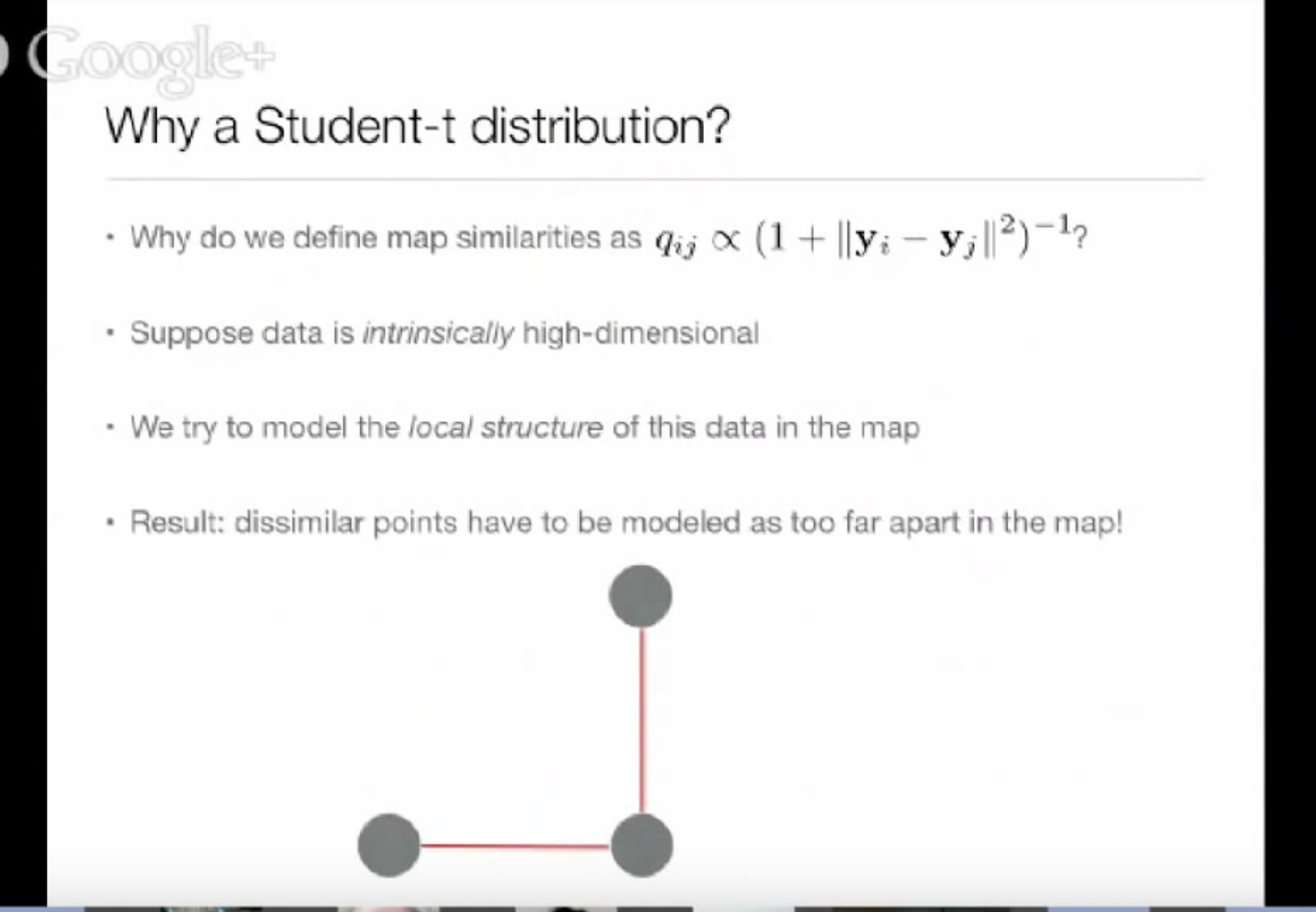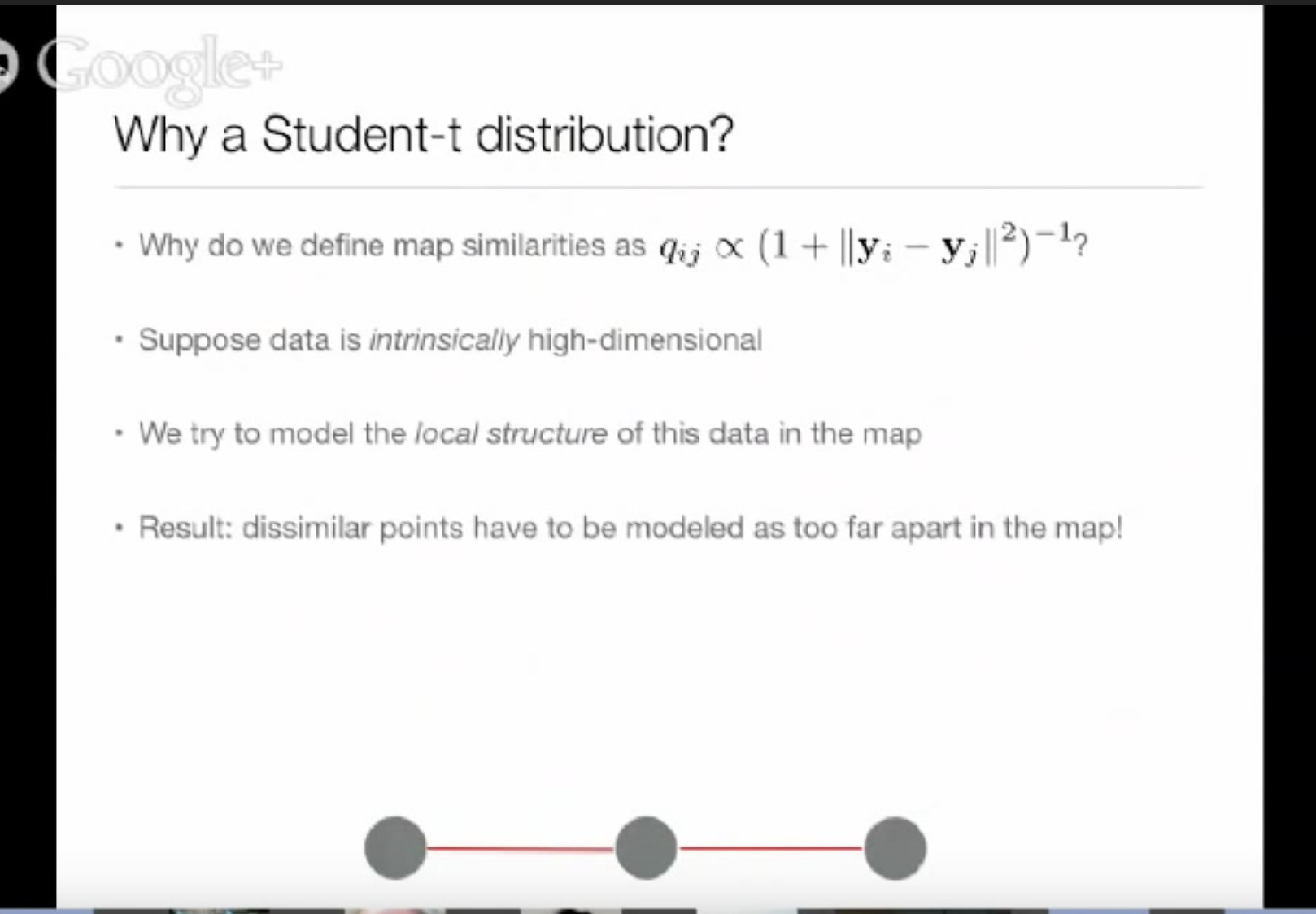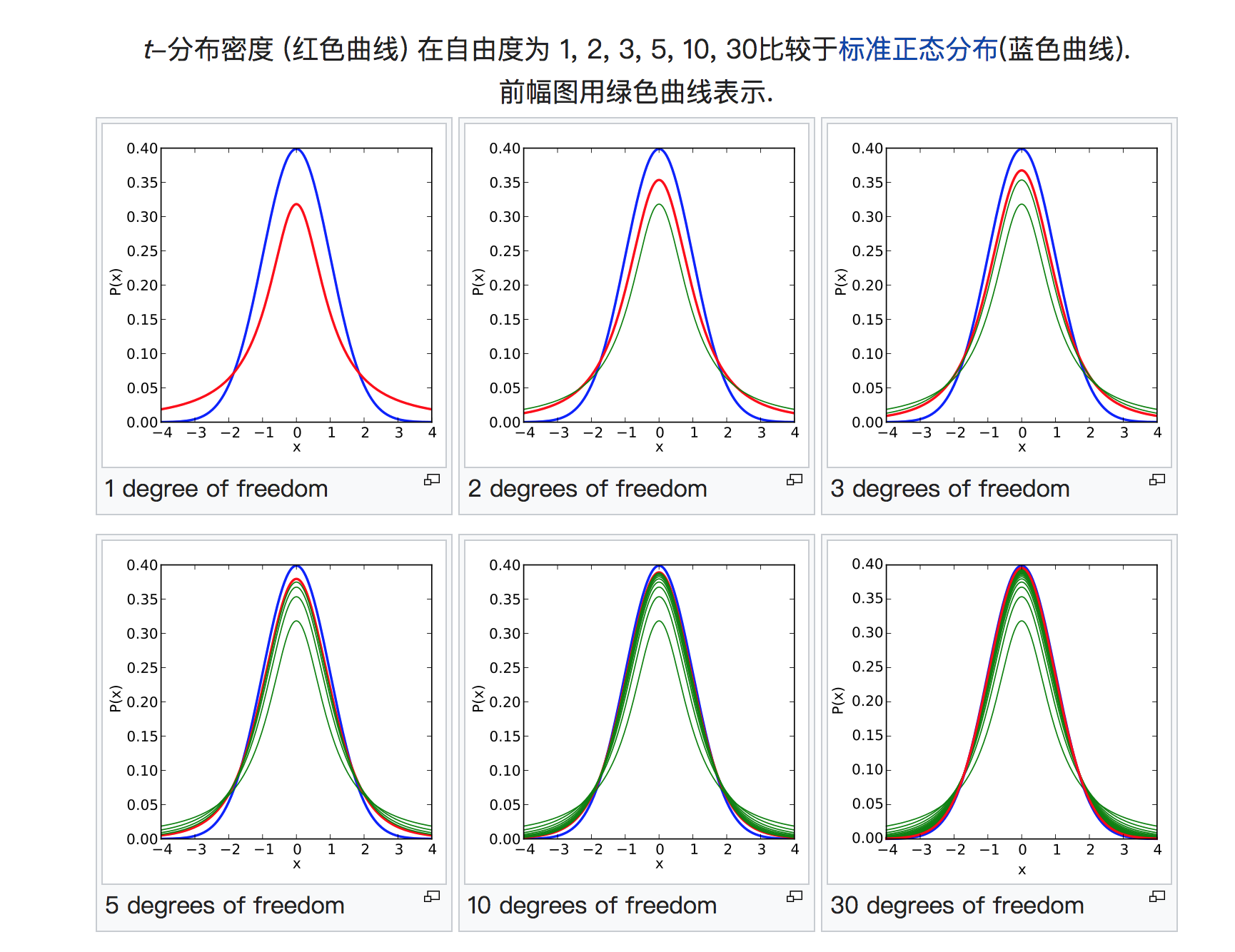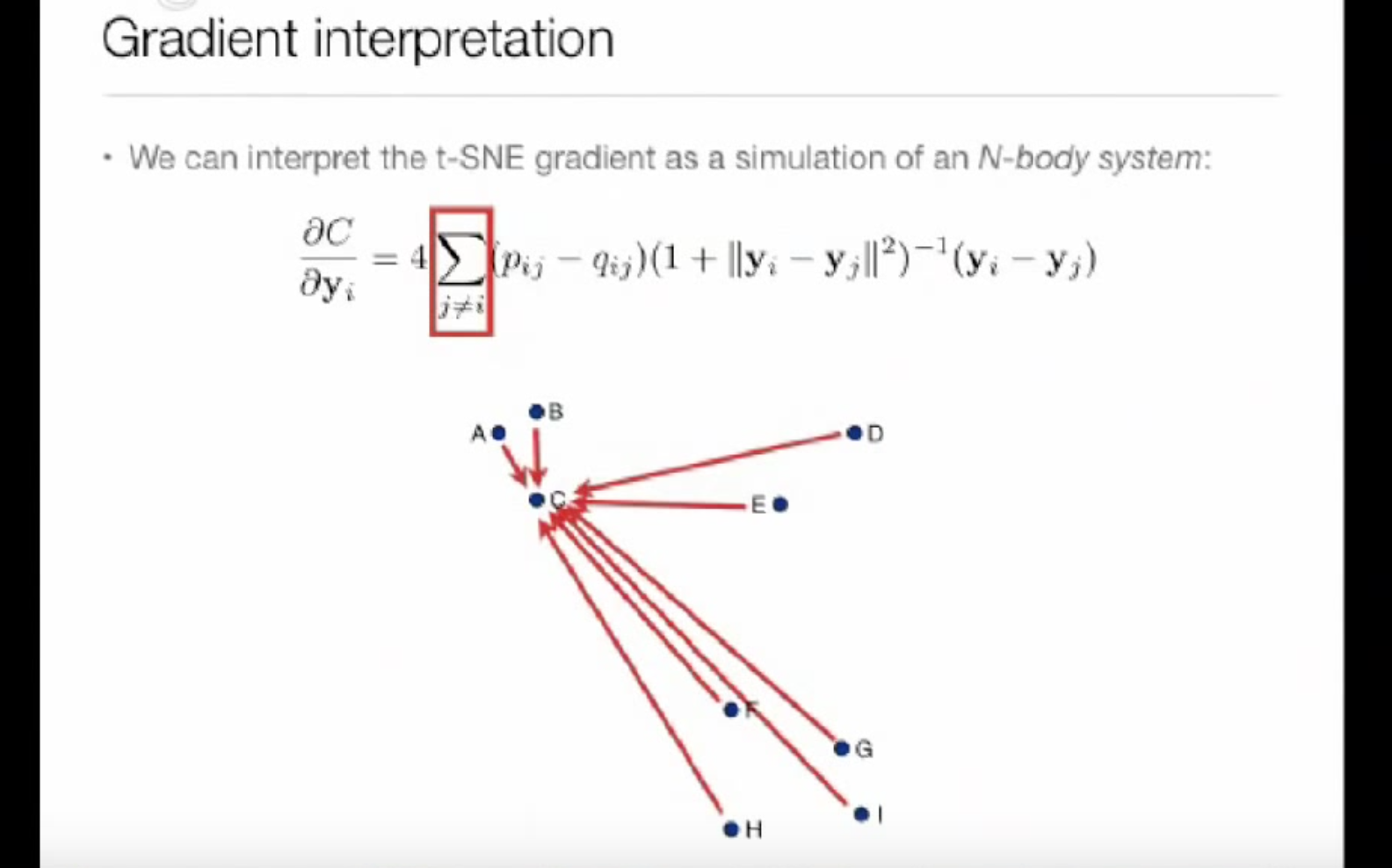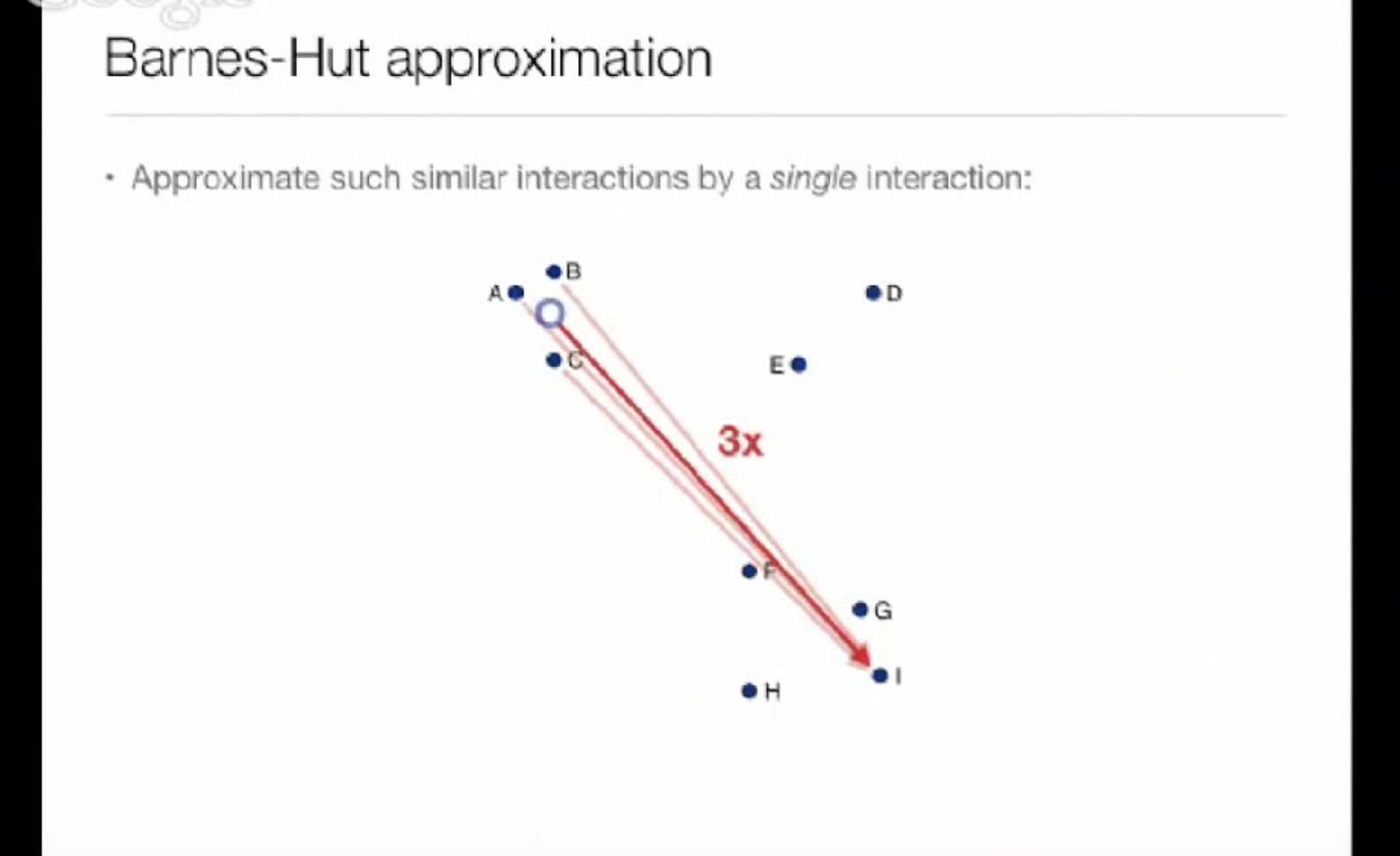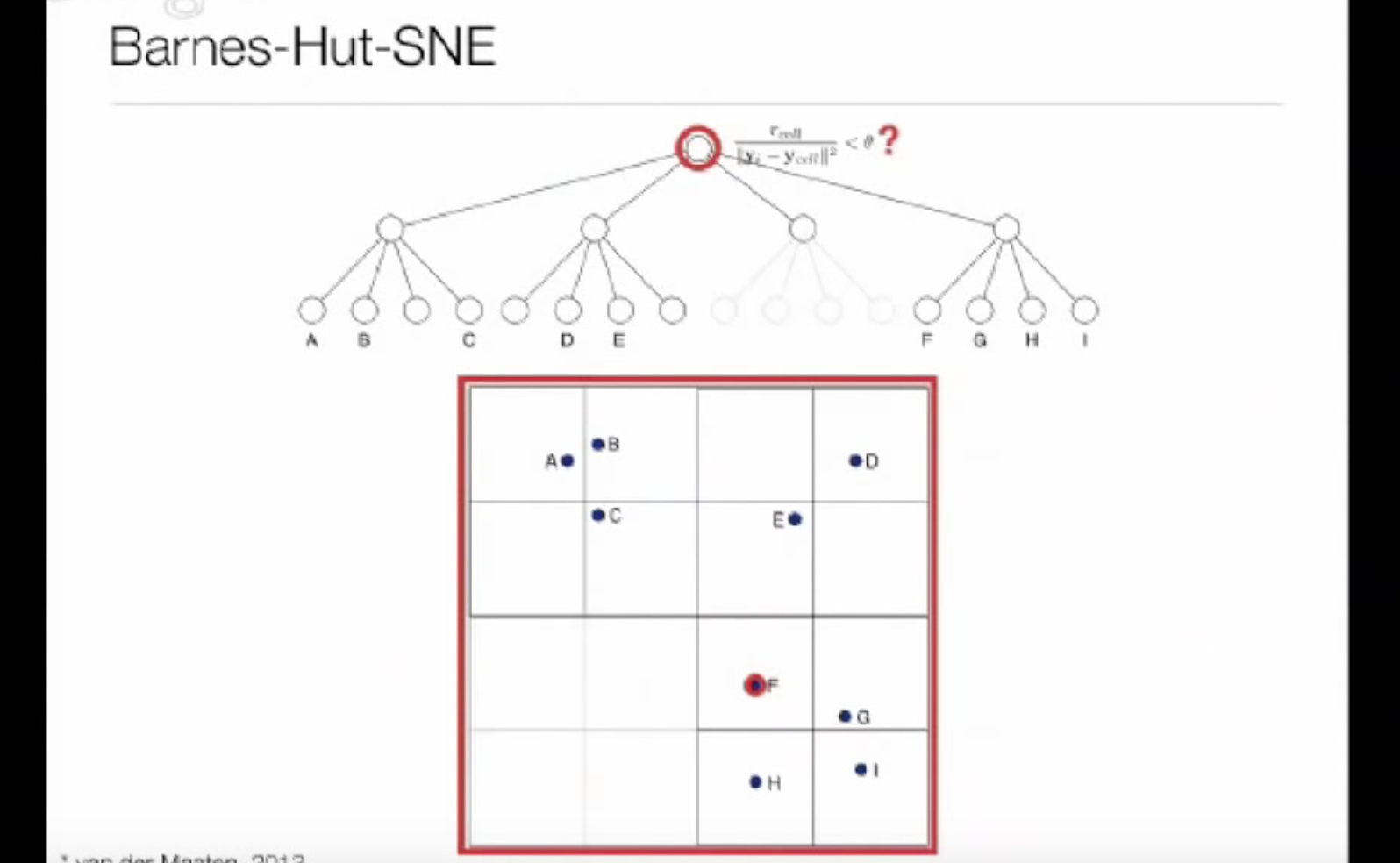sklearn裡對於binary search計算聯合分佈下面的(

t-SNE – Laurens van der Maaten理解TSNE算法t-SNE 是一种数据可视化工具，它可以将高维数据降维到2-3维以用于画图，局部相似性被这种embedding所保留。

t-SNE把原空间的数据点之间的距离转换为高斯分布概率，如果两点在高维空间距离越近，那么这个概率值越大。注意到高斯分布的这个标准差\$sigma_i\$ 对每个点都是不同的，这也是算法的创新点之一，因为理论上空间不同位置的点的密度是不同的，条件概率如此计算，

\$\$p_{j|i} = frac{exp{(-d(boldsymbol{x}_i, boldsymbol{x}j) / (2 sigma_i^2)})}{sum{i neq k} exp{(-d(boldsymbol{x}_i, boldsymbol{x}k) / (2 sigma_i^2)})}, quad p{i|i} = 0,\$\$\$\$p_{ij} = frac{p_{j|i} + p_{i|j}}{2N}.\$\$

\$\$q_{ij} = frac{(1 + ||boldsymbol{y}_i – boldsymbol{y}j)||^2)^{-1}}{sum{k neq l} (1 + ||boldsymbol{y}_k – boldsymbol{y}_l)||^2)^{-1}},\$\$\$\$KL(P|Q) = sum_{i neq j} p_{ij} log frac{p_{ij}}{q_{ij}}\$\$